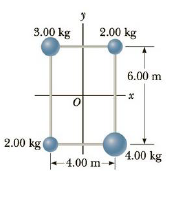Chapter 8, Problem 37P

Chapter
Section
Textbook Problem

Four objects are held in position at the corners of a rectangle by light rods as shown in Figure P8.37. Find the moment of inertia of the system about (a) the x-axis, (b) they-axis, and (c) an axis through O and perpendicular to the page.(a)

To determine
The moment of inertia of the system about x -axis.

Explanation
In general, the moment of inertia of the objects is defined as I=miri2 and in the same way, this problem is solved.

Given info The masses m1 , m2 , m3 , and m4 of the objects  are 2.00kg , 3.00kg , 4.00kg , and 2.00kg respectively and the distance of these objects from the x -axis is 3.00m .

The formula for the moment of inertia of these objects about x -axis is,

Ix=m1y2+m2y2+m3y2+m4y2

• m1 , m2 , m3 , and m4 are masses of the objects.
• y is the distance of the object from x -axis.

Substitute 2

(b)

To determine
The moment of inertia of the system about y -axis.

(c)

To determine
The moment of inertia of the system about an axis through O -and perpendicular to the page.

Still sussing out bartleby?

Check out a sample textbook solution.

See a sample solution

The Solution to Your Study Problems

Bartleby provides explanations to thousands of textbook problems written by our experts, many with advanced degrees!

Get Started